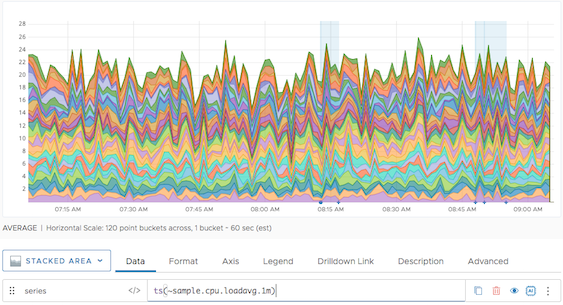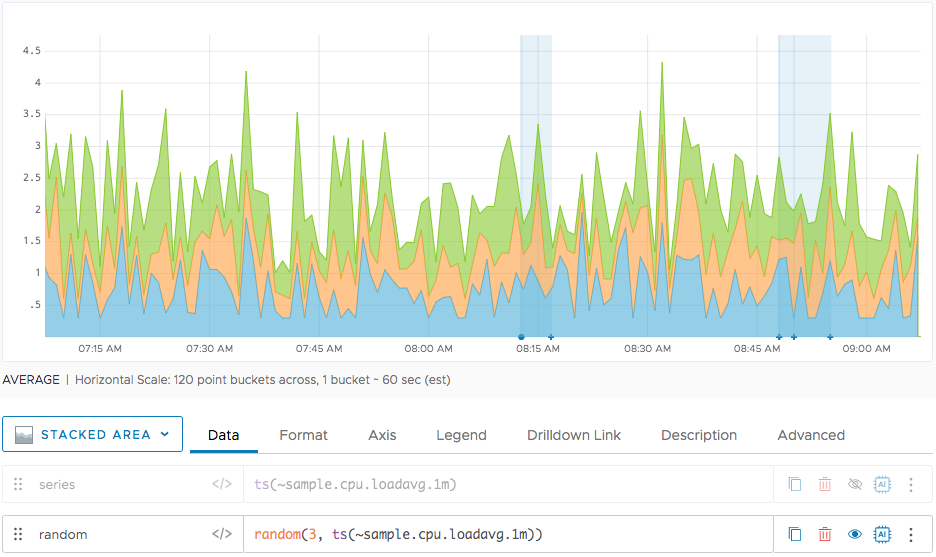Reference to the random() function

## Summary

``````random(<numberOfTimeSeries>, <tsExpression>)

random()
``````

You can use `random()` with time series and with no parameters.

 Time series filtering function Returns the specified number of time series chosen randomly from the series described by `tsExpression`. Value generator function Returns a series of random values between 0.0 and 1.0.

## Parameters

### Time-Series Filtering Function

ParameterDescription
numberOfTimeSeries Number of time series in the random subset. You can express this parameter as a number (e.g. 10) or a percentage (e.g. 17%).
tsExpression Expression that describes the time series you want to filter.

## Description

You can use `random()`:

• With time series as a filtering function.
• With no parameters as a value generator function.

### Time-Series Filtering Function

The `random()` filtering function returns the specified number of time series chosen randomly from the time series described by the `tsExpression`. Repeated calls display different random subsets.

### Value Generator

The `random()` value generator returns a random value between 0.0 and 1.0 for every point in time on a chart. Repeated calls display different random values at each point.

## Example

### Time-Series Filtering Function

The following example shows first the result of a query that returns a fairly large number of time series.The following example chart shows how we use `random()` to pick 3 of the time series. Notice how the sources that were selected are not related.